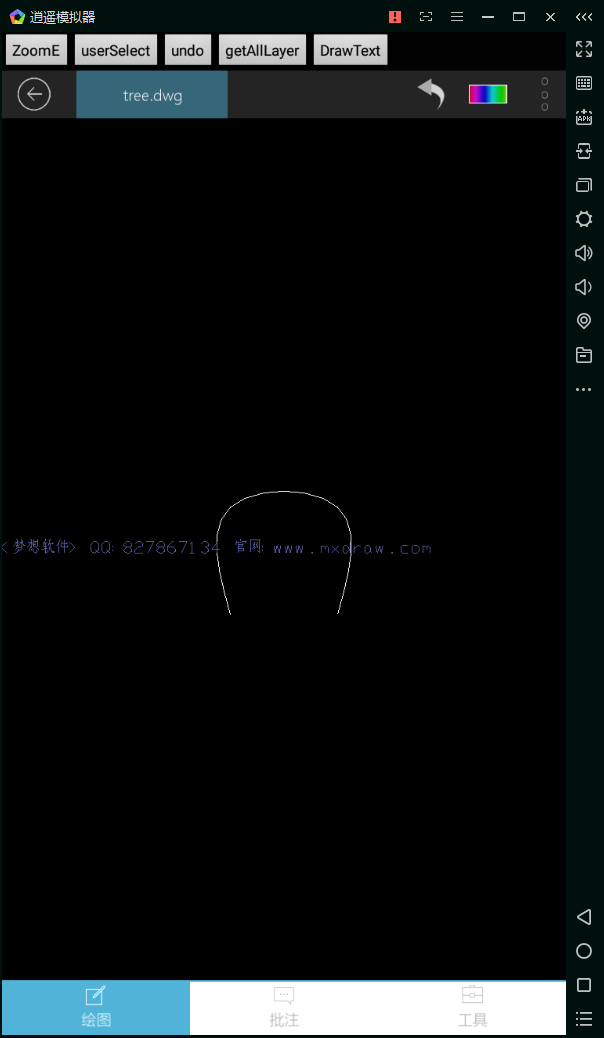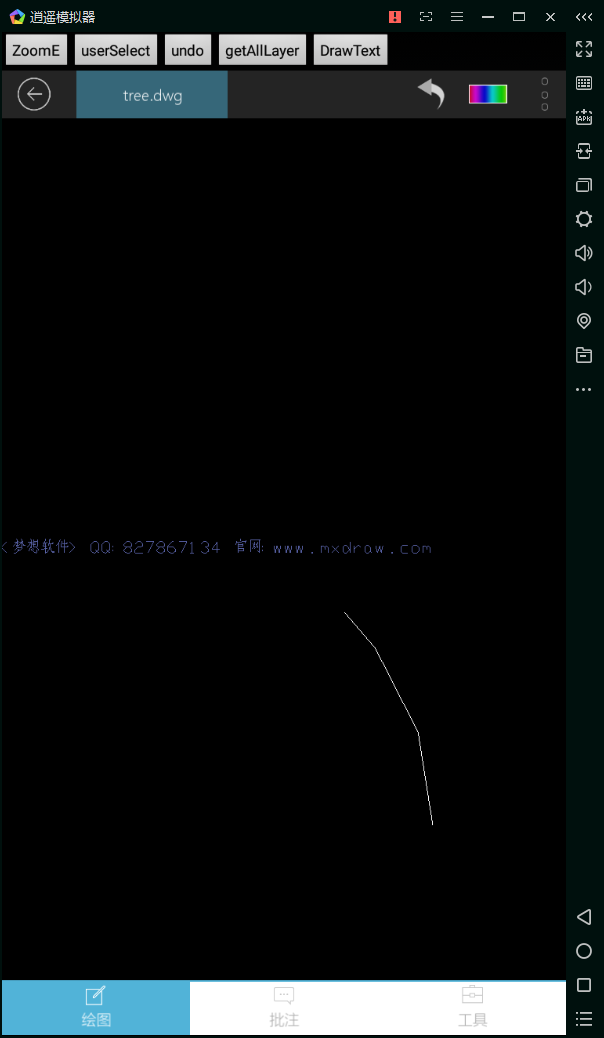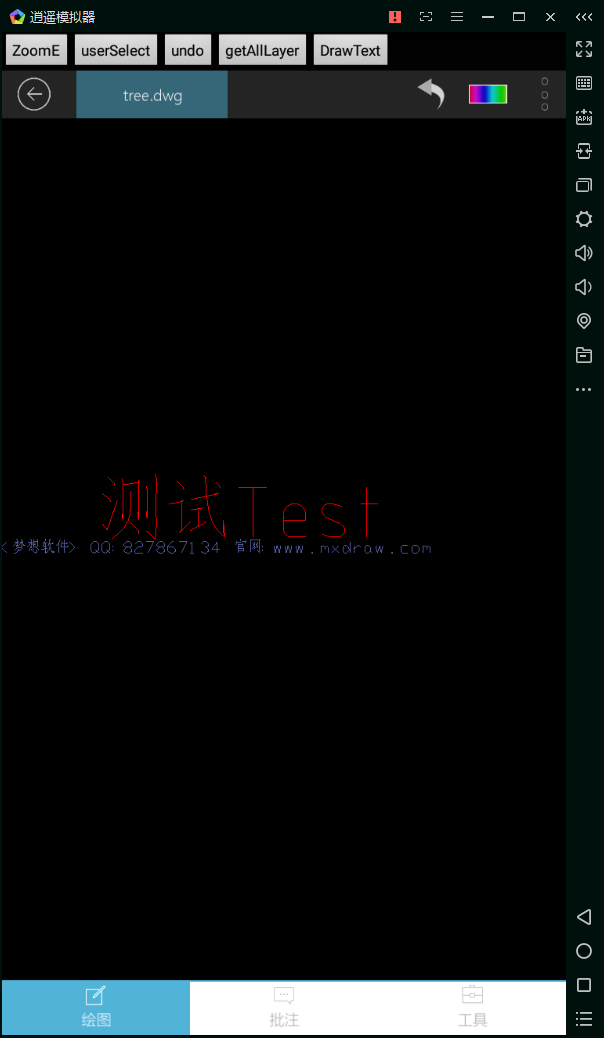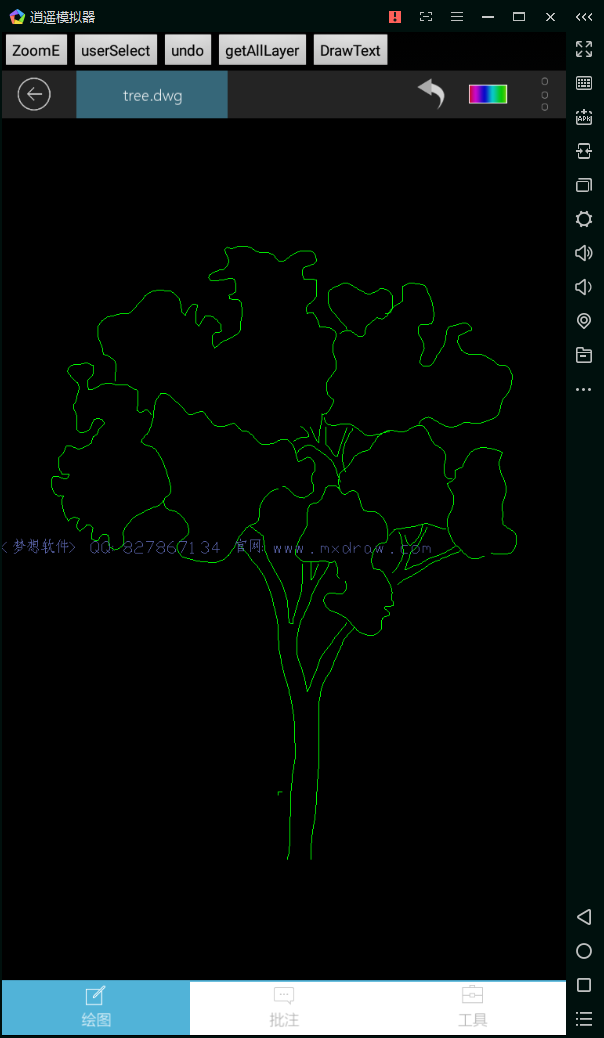MxLibDraw.drawLine

double dDx1

double dDy1

double dDx2

double dDy2

String pszName

String pszLineDefine

float dScale

```MxFunction.openFile("");

// 设置画图颜色.
long[] rgb = new long;
rgb = 255;
rgb = 0;
rgb = 0;

MxLibDraw.setDrawColor(rgb);

MxLibDraw.setLineWidth(10);

MxLibDraw.drawLine(10,10,200,300);

MxLibDraw.setLineType("MyLine");
MxLibDraw.setLineWidth(5);

MxLibDraw.drawLine(10,300,200,10);

MxFunction.zoomAll();```MxLibDraw.pathMoveTo

double dX

double dY

MxLibDraw.pathLineTo

double dX

double dY

MxLibDraw.drawPathToPolyline

```MxFunction.openFile("");
MxLibDraw.pathMoveTo(10,10);
MxLibDraw.pathLineTo(10,20);
MxLibDraw.pathLineTo(20,20);
MxLibDraw.pathLineTo(20,10);
MxLibDraw.drawPathToPolyline();
MxFunction.zoomAll();```MxLibDraw.pathMoveTo

double dX

double dY

MxLibDraw.pathLineTo

double dX

double dY

MxLibDraw.drawPathToSpline

```MxFunction.openFile("");
MxLibDraw.pathMoveTo(10,10);
MxLibDraw.pathLineTo(10,20);
MxLibDraw.pathLineTo(20,20);
MxLibDraw.pathLineTo(20,10);
MxLibDraw.drawPathToSpline();
MxFunction.zoomAll();```MxLibDraw.drawCircle

double dCenterX

double dCenterY

```MxFunction.openFile("");

MxLibDraw.drawCircle(10,10,100);

MxFunction.zoomAll();```MxLibDraw.drawArc

double dCenterX

double dCenterY

double dStartAng

double dEndAng

```MxFunction.openFile("");
MxLibDraw.drawArc(10,10,200,0,45 * 3.14159265/ 180.0);
MxFunction.zoomAll();```MxLibDraw.drawEllipse

double dCenterX

double dCenterY

double dMajorAxisX

double dMajorAxisY

```MxFunction.newFile();

MxLibDraw.drawEllipse(0,0,100,100,0.5);

MxFunction.zoomAll();```_DMxDrawX::DrawEllipseArc

DOUBLE dCenterX

DOUBLE dCenterY

DOUBLE dMajorAxisX

DOUBLE dMajorAxisY

DOUBLE dStartAng

DOUBLE dEndAngMxLibDraw.drawText

double dDx

double dDy

double height

String sTxt

`MxLibDraw.drawText(193,198,500,"测试Test");`MxFunction.drawImageMarkEx

String imageFile

double dDx

double dDy

double dScale

int iAttachment

```MxFunction.openCurrentLayer();
MxFunction.drawImageMarkEx("location2.png",19354.596193,19813.267774,0.5,MxFunction.ImageAttachment.kBottomCenter);```MxLibDraw.drawBlockReference

double dPosX

double dPosY

String pszBlkName

double dScale

double dAng

```MxFunction.newFile();

Log.e("isModifyed",MxFunction.isModifyed() ? "Y" : "N");

String sFileName = MxFunction.getWorkDir() + "/tree.dwg";
String sBlkName = "tree";
MxLibDraw.insertBlock(sFileName,sBlkName);

// drawBlockReference(double dPosX, double dPosY, String pszBlkName, double dScale, double dAng);
MxLibDraw.drawBlockReference(0,0,sBlkName,1,0);

MxFunction.zoomAll();```